# Introduction

Analog 4–20 mA current loops are one of the most used communication buses in industrial environments, where environmental variables, process sensing, and control is required. This bus type is named after its maximum and minimum current values across the line; 4 mA and 20 mA. Current loops are used not only for receiving information from sensors and field instrumentation, they are also used for transmitting control signals to actuators or other devices to regulate a controlled action. Current loops, such as 4–20 mA, have been used since the 1950s in many industrial applications. The bus is appealing due to the low implementation cost, high noise immunity due to the non-voltage dependence, and the ability to be used effectively over long distances. These characteristics are the main reasons for using a 4–20 mA loop for signaling and control. Because of this, there are several industrial sensors which implement a 4–20 mA output to indicate their measurements. Field instrumentation sensors such as pressure sensors, temperature sensors, level or flow sensors, pH sensors, and gas sensors implement a 4–20 mA connection to their outputs. Also, there are several actuators, such as valves, which can be controlled via a 4–20 mA loop. There are several different brands of commercial IC’s that implement a 4–20 mA bus receiver. In this application note, a GreenPAK™ is designed as an oxygen sensor with a 4–20 mA output, and is used to detect dangerous concentrations of oxygen for human beings in industrial environments. The app note implements the analog measurement and digital logic required to implement an integrated concentration-level alarm. This application also has a configurable limit value to detect out-of-level concentrations. To implement this 4–20 mA bus receiver, a SLG46621V is used.

The complete design file is available here. It was programmed in the GreenPAK Designer software (a part of Go Configure™ Software Hub) which is a free-to-use GUI-based program aimed to create circuit designs.

# 4–20 mA Output General Characteristics

As described before, in industrial and noisy environments, analog 4–20 mA current loops are one of the most used buses for electronic measurement and control. These buses are used not only for controlling valves or other actuators, they are also used for transmitting sensor information from field instrumentation to the control center. The main advantages of the 4–20 mA current loop are: — The data transmission is not affected by the voltage drop over the interconnecting wiring, meaning it can be used over long distances with much less degradation of the signal. — It allows the system to implement a self-monitoring routine, because currents less than 4 mA and higher than 20 mA can indicate a fault in the circuit — The 4–20 mA current loop, often, is used also to power the remote device. — The “live” or “elevated” zero of 4 mA allows powering of the device even with no process signal output from the field transmitter. The 4–20 mA communication bus is based on the principles of the Kirchhoff’s current law, which states that the sum of currents flowing toward a point is equal to the sum of currents flowing away from that point. With this principle, all current present at the beginning of a loop must reach the end. This law is the basic principle to explain the behavior of the 4–20 mA loop. The current at any position of the system can be measured and found to be the same result. This is also the main reason for using current loops with low impedance within industrial applications; they provide the benefit of obtaining a longer data path with better noise immunity. A scheme of a 4–20 mA loop can be seen in Figure 1.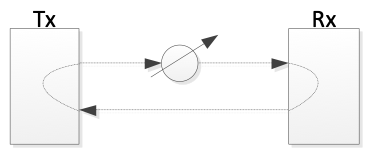Figure 1: 4–20 mA Loop Schematic

One of the most commonly used and simple circuits for measuring signals of a 4–20 mA current loop is the shunt resistor circuit. This circuit is based on Ohm’s Law. The selection of the sense resistor value is made by considering the maximum voltage that our reception device can deal with, assuming that the sense resistor is much lower than 200 kΩ. With the maximum voltage known, the resistor is then calculated by the following expression:

In order to not load the interface circuit, it’s recommended to implement an electronic buffer based on an operation amplifier. An electronic circuit that is commonly used for 4–20 mA interface to digital devices with analog inputs is shown in Figure 2.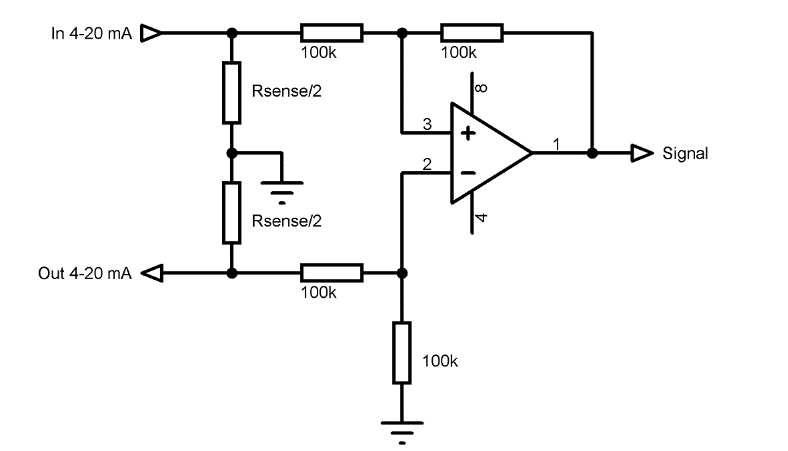Figure 2: Schematic Diagram of Electronic Circuit Interface

The shunt resistor is divided in two half-sections. This allows the circuit to prevent the voltage becoming higher than the desired values by fixing the intermediate voltage of the shunt resistor to a local ground. The differential shunt resistor voltage is converted to a single ended voltage with the operational amplifier having a differential amplifier configuration at unity gain. Resistor values of the operational amplifier circuit are selected to not load the current loop.

# Schematic Diagram

To show how a GreenPAK can be used for interfacing a 4–20 mA bus, this app note implements an oxygen concentration level alarm useful for industrial applications. In some environments, detecting dangerous oxygen concentrations for human beings is an important safety task. As everyone knows, oxygen is a vital gas and a typical oxygen concentration is 20.9% v/v. If an environment has an oxygen concentration lower than 19.5 % v/v, it can be considered as an environment with oxygen deficiency. Oxygen concentrations lower than 16 % v/v is unsafe for human beings. Additionally, higher concentrations of oxygen can also be a risk for humans. If the oxygen concentration rises, material and gas inflammability increase. As an example, if the oxygen concentration is higher than 24 % v/v, some elements such as clothes can start burning spontaneously. To implement an oxygen concentration alarm, a sensor from Honeywell is used. The MF020–2-LC3 sensor has a measuring range from 0.1 % v/v to 25 % v/v with a 4–20 mA analog output and a power supply of 24 VDC. To measure the sensor output with a Dialog SLG46621 CMIC, the Programmable Gain Amplifier (PGA) and Analog to Digital Converter (ADC) of the IC are used. To do this, the maximum input voltage of the analog converter must be considered. If the ADC is used with an internal voltage reference of 1.2 V, and with unity gain, the maximum input voltage for the converter is 1 V. By applying the RSENSE expression shown in previous section, the resistor value needed for the shunt is:

With the selected RSENSE, the output voltages that will be obtained when the current loop has 4 mA and 20 mA are 200 mV and 1 V respectively. The minimum voltage level is compliant with the minimum input voltage to the SLG46621 ADC, which is 30 mV. In Figure 3, the electronic circuit that must be used for interfacing the MF020–2-LC3 sensor to the SLG46621 is shown. The operational amplifier used for this application is the SLG88103.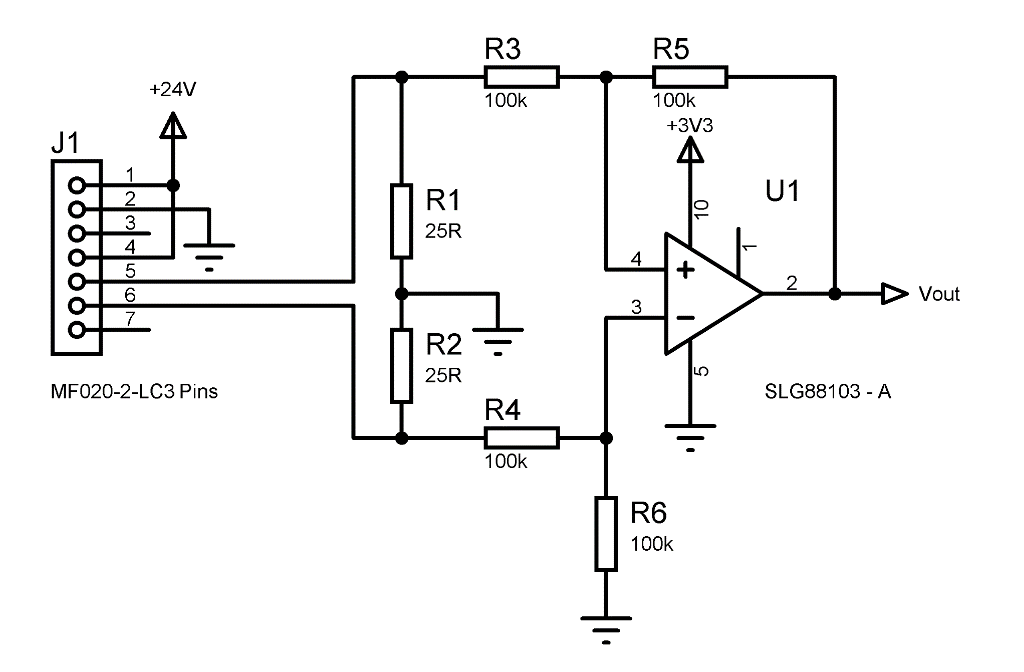Figure 3: Schematic Diagram of Sensor Interface

The VOUT signal of the 4–20 mA output is connected to the Analog input of the SLG46621 chip, as seen in Figure 3.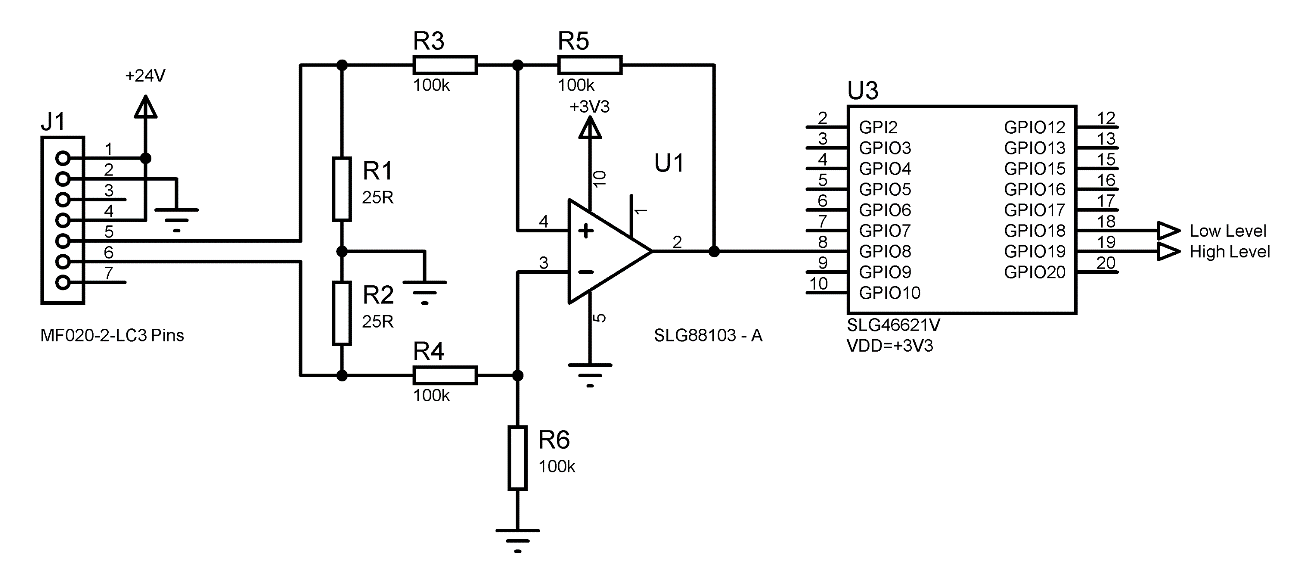Figure 4: Schematic Diagram of Sensor Interface

# Implementation

The implementation of the 4–20 mA interface for an oxygen concentration level alarm is made with a SLG46621V GreenPAK. This CMIC has one Analog to Digital Converter, and several Digital Comparators that can be used for analyzing the voltage at the input of the ADC. To configure the comparison levels, the internal comparator registers are used. A block diagram of the implementation can be seen in Figure 5.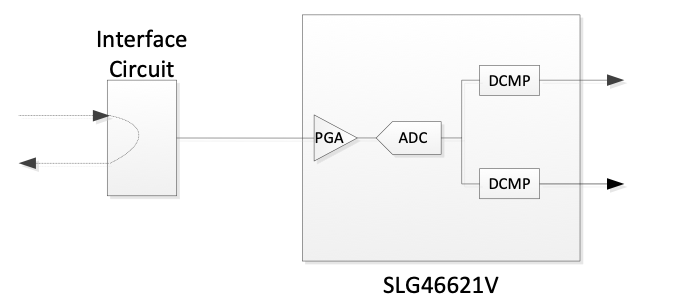Figure 5: Oxygen Concentration Level Block Diagram

The allowed upper and lower oxygen concentrations are configured in the registers. This is done by considering that the ADC has an 8-bit resolution. The maximum input voltage (1 V) is converted to 255, which corresponds to an oxygen concentration of 25% v/v. With this consideration, the comparison value that must be configured for the desired concentration can be calculated as follows.

In this case, the minimum concentration that is required is 16%, so the corresponding register must be configured to 163. The maximum concentration allowed is 22%, so the corresponding register must be configured to 225.

To implement the analog to digital conversion, the ADC and PGA of the SLG46621V must be configured. Figure 6 shows the PGA configuration and it is configured as single-end ADC with unity gain.

The PGA defines the analog input to be PIN 8 of the GreenPAK™. The ADC complementary configuration defines the internal RC oscillator as the clock source and the internal 1.2 V regulator as the voltage reference. This can be seen in Figure 7.

The ADC output is compared with the configured values in the DCMP0 and DCMP1. In Figure 8 and Figure 9 the configuration of the DCMP’s can be seen. They are configured to compare the ADC output value with the internal registers.

The OUT+ output of each digital comparator will be high when the IN+ input of the comparator is higher than the IN- input. In this case, the DCMP0 OUT+ output will be high when the ADC is higher than the configured value. This comparator is used as the high-level detector, so this OUT+ signal is connected directly to PIN 19 and the Register 0 is configured to 225. The DCMP1 OUT+ output will be high when the ADC is higher than the configured value. Because this DCMP is used as the low-level detector, the OUT+ output must be inverted to be connected to PIN 18. This is done with 2-bit LUT4, whose configuration is shown on Figure 10. This DCMP compares the ADC conversion with the value configured on Register 3.

Pins 18 and 19 are the level detector outputs, representing the low-level detector and the high-level detector respectively. Pin 8 is the analog input. On Figure 11, Figure 12 and Figure 13, their configuration is shown.

The entire Oxygen Level Concentration alarm implementation is shown in Figure 14 and Figure 15, where Matrix 0 and Matrix 1 of the Dialog SLG46621V GreenPAK are shown.

# Testing

To test the implementation, a simulated 4–20 mA output of the oxygen sensor was made. This output has the full range of the sensor output and it’s represented as a triangular wave. The current is linearly increased and decreased to show the change in oxygen concentration. The simulated signal was connected to the interface circuit so it can be measured with the SLG46621V. To analyze the results, pins 18 and 19 were logged with an oscilloscope. In Figure 16, the simulated sensor output can be seen with the low-level indication of the SLG46621V. It shows how the output of pin 18 holds low when the sensor output is lower than the configured value.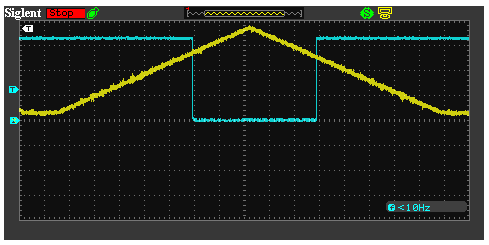Figure 16: Pin 18 Oxygen Concentration Low-Level Detection

In Figure 17, the simulated sensor output can be seen with the high-level indication of the SLG46621V. It shows how the output of pin 19 holds high when the sensor output is higher than the configured value.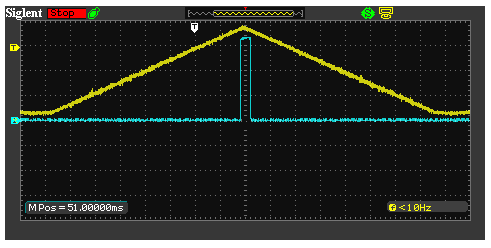Figure 17: Pin 19 Oxygen Concentration High-Level Detection

# Conclusion

In this application note, we implemented a 4–20 mA interface for measuring the output of an oxygen sensor to detect out-of-range oxygen concentrations. 4–20 mA current loops are used in most industrial sensors and actuators because of the buses’ high noise immunity and compatibility with long distance wiring. There are several methods of interfacing to a 4–20 mA output. In this application note, one of the simplest and most commonly used methods of interpreting a 4–20 mA line is shown. It is also described how the signal can be conditioned to be measured with a Dialog GreenPAK by using the integrated Analog to Digital Converter. The size of the OpAmps and GreenPAK measurement system is smaller than many other implementations, and it can be further reduced by incorporating more of the logic for the specific application into the SLG46621V.

--

--